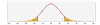# Confidence Interval For Two Variances

Confidence interval calculator for the ratio of two variances with calculation steps.#### Two variances confidence interval calculator

When using the sample data, we know the sample ratio between the variances, but we don't know the ratio's true value. We may treat the population's proportions as random variables and calculate the confidence interval.
First, we need to define the confidence level, which is the required certainty level that the true value will be in the confidence interval. Researchers commonly use a confidence level of 0.95.
We use the F distribution.

##### Confidence interval for two variances formula
 [ Fα/2(n₂-1, n₁-1)* S²₁ , F1-α/2(n₂-1, n₁-1)* S²₁ ] S²₂ S²₂
##### How to use the confidence interval calculator for two variances?
1. Data is:
S₁, S₂, n₁, n₂ - enter the sample standard deviations (S₁, S₂), and the sample sizes (n₁, n₂).
S²₁, S²₂, n₁, n₂ - enter the sample variances (S²₁, S²₂), and the sample sizes.
Raw data - enter the delimited data, separated by a comma, space, or enter. In this case, the tool will calculate the variances and the sample size.
2. Confidence level - The certainty level that the true value of the estimated parameter will be in the confidence interval, usually 0.95.
3. Rounding - how to round the results?
When a resulting value is larger than one, the tool rounds it, but when a resulting value is less than one the tool displays the significant figures.
4. Sample sizes (n1, n2) - the number of subjects.
5. Sample Variances (S²₁, S²₂) or sample standard deviations (S₁, S₂):
6. Bounds:
Two-sided: the confidence interval is between the lower bound, and the upper bound.
Upper bound: the confidence interval is between zero and the upper bound.
Lower bound: the confidence interval is between the lower bound, and infinity.

The confidence interval is also equivalent to the F test for equality of two variances:
Confidence interval with upper bound is equivalent to left tail test.
Confidence interval with lower bound is equivalent to right tail test.
The directions of the one-sided test and one-sided confidence interval are opposite, and the reason is that you check different directions: In a confidence interval, you may check if the expected value falls inside the confidence interval that is built around the estimated value.
In a statistical test, you check if the estimated value falls inside the region of acceptance that is built around the expected value.